In this page, we are providing Online Education Light Reflection and Refraction Class 10 Extra Questions and Answers Science Chapter 10 pdf download. NCERT Extra Questions for Class 10 Science Chapter 10 Light Reflection and Refraction with Answers will help to score more marks in your CBSE Board Exams.

## Online Education for Class 10 Science Chapter 10 Extra Questions and Answers Light Reflection and Refraction

Extra Questions for Class 10 Science Chapter 10 Light Reflection and Refraction with Answers Solutions

### Extra Questions for Class 10 Science Chapter 10 Very Short Answer Type

Light Class 10 Extra Questions Question 1.
Draw a schematic representation of different type of mirrors.
Types of mirror:

1. Plane mirror
2. Spherical mirror
(i) concave mirror
(ii) convex mirror

Plane mirror: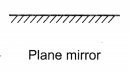(i) concave mirror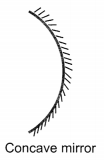(ii) convex mirror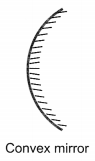Light Extra Questions Class 10 Question 2.
Define focal length.
Focal length (f): The distance between the pole and principal focus (F) of a spherical mirror is called the focal length of the mirror. It is denoted by f.
f = $$\frac{R}{2}$$Light Reflection And Refraction Class 10 Extra Questions Question 3.
Define one dioptre.
1 dioptre is the power of a lens whose focal length is 1 metre. 1 D = 1 m-1

Chapter 10 Science Class 10 Extra Questions Question 4.
Define focus.
Principal focus: A point on the principal axis of a spherical mirror where the rays of light parallel to the principal axis meet or appear to meet after reflection from the spherical mirror is called principal focus.

Extra Questions Of Light Class 10 Question 5.
What is concave and convex mirror?

• Concave mirror: A spherical mirror, whose reflecting surface is curved inwards, that is it faces towards the centre of the sphere, is called a concave mirror.
• Convex mirror: A spherical mirror whose reflecting surface is curved outwards, is called a convex mirror.

Class 10 Light Extra Questions Question 6.
Define
1. Reflection of light
2. Beam of light
(1) Reflection: When light falls on a surface and bounces back to the medium, the phenomena is called reflection.
(2) Beam: A beam is a bundle of rays, which originates from a common source and travels in the same direction.

Light Reflection And Refraction Extra Questions Question 7.
Define light and write its properties.
Light: It is a form of energy which produces the sensation of sight.

• Light exhibits dual nature i.e., wave as well as particle nature.
• It travels with speed of 3 × 108 m/s in vacuum. However, speed is inversely proportional to optical density of medium.

Extra Questions On Light Class 10 Question 8.
Draw a ray diagram showing the path of rays of light when it enters with oblique incidence (i) from air into water.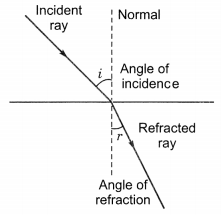### Extra Questions for Class 10 Science Chapter 10 Short Answer Type I

Class 10 Science Chapter 10 Extra Questions Question 1.
Draw the ray diagrams showing the image formation by a concave lens.

 Position of the object Position of the image Relative size of the image Nature of the image At infinity At focus F1 Highly diminished point sized Virtual and erect Between infinity and optical centre O of the lens Between focus F and optical centre O Diminished Virtual and erect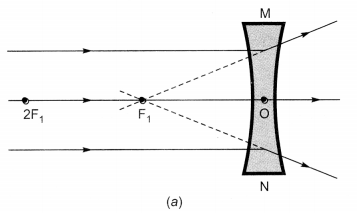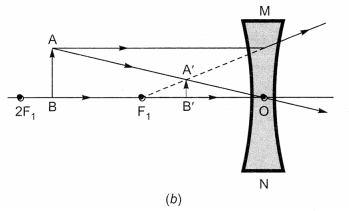Nature, position and relative size of the image formed by a concave lens

Ncert Class 10 Science Light Reflection And Refraction Extra Questions Question 2.
Write lens formula and define magnification.
Lens formula and magnification
$$\frac { 1 }{ v }-\frac { 1 }{ u }=\frac { 1 }{ f }$$
u = object distance
v = image distance
f = focal length

Magnification (m): Magnification is defined as the ratio of height of image and to height of the object.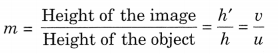h’ = height of image
h = height of object

Class 10 Light Reflection And Refraction Extra Questions Question 3.
State laws of reflection.
Laws:

1. The angle of incidence is equal to the angle of reflection.
2. The incident ray, the normal to the mirror at the point of incidence and reflected ray, all lie in the same plane.

These laws of reflection are applicable to all types of reflecting surfaces including spherical surfaces.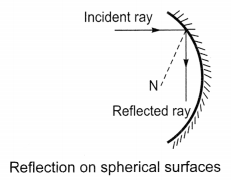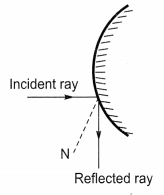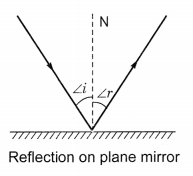Class 10 Science Light Reflection And Refraction Extra Questions Question 4.
Write four difference between real and virtual image.
Real image:

1. When rays of light after reflection meets at a point real image is formed.
2. Real image can be obtained on screen.
3. Real image is formed in front of mirror.
4. Real image is always inverted.

Virtual image:

1. When rays of light do not actually meet but appear to meet at a point after reflection, virtual image is formed.
2. Virtual image cannot be obtained on screen.
3. Virtual image is formed behind the mirror.
4. Virtual image is always erect.

Class 10 Science Ch 10 Extra Questions Question 5.
If the speed of light in vacuum is 3 × 108 ms-1, find the speed of light in a medium of absolute refractive index 1.5.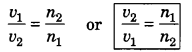Here, v1 = 3 × 108 m/s, n1 = 1, n2 = 1.5
v2 = $$\frac { 1 }{ 1.5 }$$ × 3 × 108
v2 = 2 × 108 m/s

Light Chapter Extra Questions Class 10 Question 6.
An object of height 6 cm is placed perpendicular to the principal axis of a concave lens of focal length 5 cm. Use lens formula to determine the position, size and nature of the image if the distance of the object from the lens is 10 cm.
h = 6 cm, f = -5 cm, u = -10 cm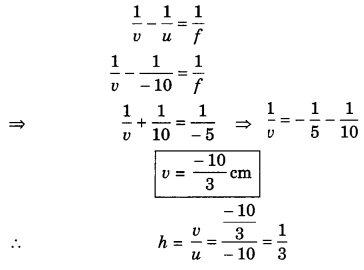Image is diminished and erect.

Extra Questions Light Class 10 Question 7.
Define refractive index and relative refractive index.
1. Refractive index: The ratio of speed of light in vacuum (c) to the speed of light in any medium (v) is called refractive index of the medium.2. Relative refractive index: The relative refractive index of a medium with respect to other medium is the ratio of the speed of light in the first medium with respect to the second medium.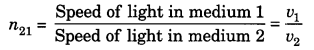Here, n21 = Relative refractive index of medium 2 with respect to medium 1 is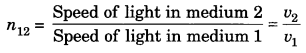Here, n12 = Relative refractive index of medium 1 with respect to medium 2.

Class 10 Chapter 10 Science Extra Questions Question 8.
Write some illustrations of refraction.
Some applications of refraction:

1. Bottom of a tank or a pond containing water appears to be raised due to refraction.
2. When a thick glass slab is placed over some printed matter the letters appear raised when viewed through the glass slab.
3. When a pencil is partly immersed in water, it appears to be bent at the interface of air and water.
4. A lemon kept in water in a glass tumbler appears to be bigger than its actual size, when viewed from the sides.

Class 10 Science Chapter Light Extra Questions Question 9.
Name the type of mirror used in solar furnace. How is high temperature achieved by this device?  (CBSE 2012)
Concave mirror is used in solar furnace. The solar furnace is placed at the focus of the large concave reflector. The concave reflector focus the Sim’s heat rays on the furnace and a high temperature is achieved.

Light Reflection And Refraction Class 10 Extra Questions And Answers Question 10.
The absolute refractive indices of glass and water are $$\frac{4}{3}$$ and $$\frac{3}{2}$$ respectively. If the speed of light in glass is 2 × 108 ms-1, calculate the speed of light in (i) vacuum and (ii) water.  (CBSE2015)
Given, µg= $$\frac{4}{3}$$ and µw = $$\frac{3}{2}$$
Speed of light in glass = 2 × 108 ms-1
(i) Speed of light in vacuum, c = µg × vg = $$\frac{4}{3}$$ × 2 × 108 = 2.67 × 108 ms-1
(ii) Speed of light in water,Extra Questions From Light Class 10 Question 11.
A convex lens of focal length 25 cm and a concave lens of focal length 10 cm are placed in closed contact with each other. Calculate the lens power of the combination.
Power of convex lens, P1 = $$\frac{1}{f_{1}}=\frac{1}{0.25}$$ = 4D
Power of concave lens, P2 = $$\frac{1}{f_{2}}=\frac{1}{-0.1}$$ = -10D
power of combination, P = P1 +P2 = 4D – 10D = -6D

### Extra Questions for Class 10 Science Chapter 10 Short Answer Type II

Class 10 Science Chapter Light Reflection And Refraction Extra Questions Question 1.
Define power of a lens. What is its unit? One student uses a lens of focal length 50 cm and another of -50 cm. What is the nature of the lens and its power used by each of them? (NCERT Exemplar)
Power of a lens: The power of a lens is defined as reciprocal of its focal length.
P = $$\frac{1}{f}$$
f= focal length (in metre)

The SI unit of power is ‘dioptre’. It is denoted by the letter D.
Here, f= 50 cm = 0.5 m
Power P = $$\frac{1}{f}=\frac{1}{0.5}$$ = +2D

f = – 50 cm = -0.5 m
Power P = $$\frac{1}{f}=\frac{1}{-0.5}$$ = -2D

Extra Questions For Class 10 Science Chapter 10 Question 2.
Define lens. What is difference between convex and concave lens?
Lens: A transparent medium bound by two surfaces, of which one or both surfaces are spherical, forms a lens:
Convex lens: A lens having two spherical surfaces, bulging outwards is called a double convex lens or convex lens.

• It is thicker at the middle as compared to the edges.
• Convex lens converges light.
Hence, convex lens are called converging lens.

Concave lens: A double concave lens is bounded by two spherical surfaces curved inwards.

• It is thicker at edges than in the middle.
• Concave lens is diverging in nature.

Class 10 Physics Chapter Light Extra Questions Question 3.
Draw ray diagrams showing the image formation by a convex mirror when an object is placed (NCERT Exemplar)
(a) at infinity
(b) at finite distance from the mirror
Formation of image by convex mirror:

 Position of the object Position of the image Relative size of the image Nature of the image At infinity At focus F behind the mirror Highly diminished, point sized Virtual and erect Between infinity and the pole P of the mirror Between P and F behind the mirror Diminished Virtual and erect

Ray diagram for image formation by convex mirror: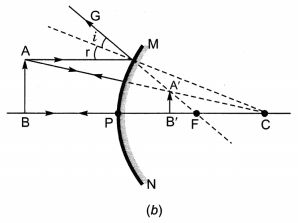Class 10 Science Light Extra Questions Question 4.
Write down the uses of concave and convex mirror.
Uses of mirrors:
1. Uses of concave mirrors:

• Concave mirrors are commonly used in torches, searchlights and vechicles headlights to get powerful beam of light.
• It is used in shaving mirrors to see large image of the face.
• Dentists use concave mirror to see large images of the teeth of patients.
• Large concave mirrors are used to concentrate sunlight to produce heat in solar furnace.

2. Uses of convex mirrors

• Convex mirrors are used as rear-view (wing) mirrors in vehicles.
• Convex mirrors are used as street reflectors because they are able to spread light over a bigger area.

Question 5.
(i) “The refractive index of kerosene is 1.44.” What is meant by this statement?
(ii) A ray of light strikes a glass slab of an angle of incidence equal to 30°. Find the refractive index of glass such that the angle of refraction is 19.5°. (Take sin 19.5° = $$\frac{1}{3}$$ and sin 30° = $$\frac{1}{2}$$ ) (CBSE 2015)
(i) Refractive index of any medium with respect to another indicated the extent to which binding of light takes place when it enters from the first medium to the given medium. The given value of refractive index also states that speed of light in kerosene is $$\frac{1}{1.44}$$ times the speed of light in a vacuum.
(ii) Given, i = 30°, r = 19.5°### Extra Questions for Class 10 Science Chapter 10 Long Answer Type

Question 1.
Write the laws of refraction. Explain the same with the help of ray diagram, when a ray of light passes through a rectangular glass slab. (NCERT Exemplar)
Laws of refraction:

• The incident ray, the refracted ray and the normal at the point of incidence, all lie in the same plane.
• The ratio of sine of angle of incidence to the sine of angle of refraction is a constant, for the light of a given colour and for the given pair of media. This law is also known as Snell’s law of refraction.

If i is the angle of incidence and r is angle of refraction.
sin i / sin r = constant

Refraction through glass slab:

• The ray of light enters from rarer to denser medium at point O that is from air to glass and bends towards the normal.
• At ‘O’, the light ray enters from glass to air, that is, from a denser medium to a rarer medium. The light here bends away from normal.
• The emergent ray is parallel to the incident ray. However the light ray shifts slightly sideward.
• Refraction is due to change in speed of light when it enters from one medium to another.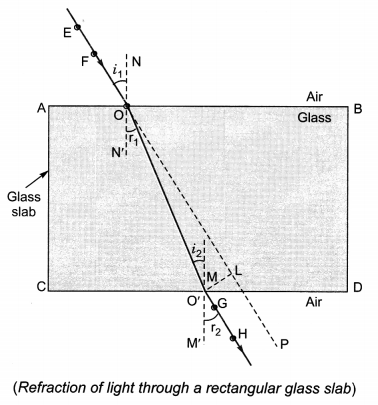Question 2.
Draw ray diagrams showing the image formation by a convex lens when an object is placed (NCERT Exemplar)
(a) between optical centre and focus of the lens
(b) between focus and twice the focal length of the lens
(c) at twice the focal length of the lens
(d) at infinity
(e) at the focus of the lens
Nature, position and relative size of the image formed by a convex lens for various positions of the object

 Position of the object Position of the image Relative size of the image Nature of the image (a) Between optical centre O and focus F1 On the same side of the lens as the object Enlarged Virtual and erect (b) Between F1 and 2F1 Beyond 2F2 Enlarged Real and inverted (c) At 2F1 At 2F2 Same size Real and inverted (d) At infinity At focus F2 Highly diminished, point-sized Real and inverted (e) At focus F1 At infinity Infinitely large or highly enlarged Real and inverted

Ray diagram for the image formation by convex lens: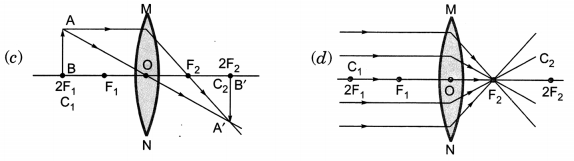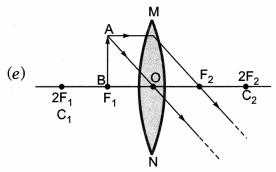The position, size and the nature of the image formed by a convex lens for various positions of the object.

Question 3.
Draw ray diagrams showing the image formation by a concave mirror when an object is placed
(a) at the focus of the lens
(b) between focus and twice the focal length of the lens
(c) beyond twice the focal length of the lens
Image formation by a concave mirror for different positions of the object

 Position of the object Position of the image Size of the image Nature of the image (a) At F At infinity Highly enlarged Real and inverted (b) Between C and F Beyond C Enlarged Real and inverted (c) Beyond C Between F and C Diminished Real and inverted

Question 4.
Draw ray diagram for the image formation by a concave mirror.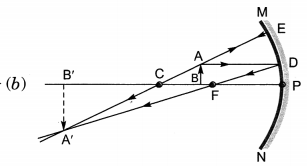Question 5.
A spherical mirror produces an image of magnification -1 on a screen placed at a distance of 50 cm from the mirror.
(a) Write the type of mirror.
(b) Find the distance of the image from the object.
(c) What is the focal length of the mirror? (CBSE 2014)
(a) Concave mirror
(b) m = -1, u = -50 cm,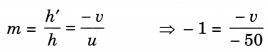∴ v = -50 cm
(c)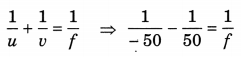∴ f = -25 cm

Question 6.
Draw a ray diagram to show the path of the reflected ray in each of the following cases. A ray of light incident on a convex mirror and concave mirror
(a) strikes at its pole making an angle θ with the principal axis.
(b) is directed towards its principal focus.
(c) is parallel to its principal axis.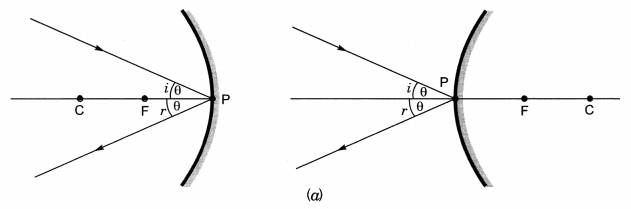### Light Reflection and Refraction HOTS Questions With Answers

Question 1.
The refractive indices of water and glass with respect to air are 4/3 and 3/2 respectively. If the speed of light in glass is 2 × 108 ms-1, find the speed of light in (i) air, (ii) water.
(i) Let v1 = speed of light in air,
v2 = speed of light in glass,
then, $$\frac{n_{2}}{n_{1}}$$ = refractive index of glass with respect to air = $$\frac{3}{2}$$
$$\frac{v_{1}}{v_{2}}=\frac{n_{2}}{n_{1}}$$ , v2 = 2 × 108 m/s
$$\frac{n_{1}}{n_{2}}$$ = $$\frac{2}{3}$$
v1 = $$\frac{3}{2}$$ × 2 × 108 m/s = 3 × 108 m/s

(ii) Let = speed of light in water,
v2 = speed of light in air,
then $$\frac{n_{2}}{n_{1}}$$ = refractive index of water with respect to air
$$\frac{v_{1}}{v_{2}}=\frac{n_{2}}{n_{1}}$$ Here, u2 = 3 × 108 m/s
= $$\frac{n_{1}}{n_{2}}$$ = $$\frac{4}{3}$$
v1 = $$\frac{3}{4}$$ × 3 × 108 = 1.5 × 108 m/s.

Question 2.
The image of a candle flame formed by a lens is obtained on a screen placed on the other side of the lens. If the image is three times the size of the flame and the distance between lens and image is 80 cm, at what distance should the candle be placed from the lens? What is the nature of the image at a distance of 80 cm and the lens? (NCERT Exemplar)
m = $$\frac{v}{u}$$ = – 3,  v = +80 cm[m is negative since image is real (obtained on a screen)]
$$\frac{v}{u}$$ = -3Image is real (obtained on screen), inverted and enlarged. The lens is convex.

Question 3.
Size of image of an object by a mirror having a focal length of 20 cm is observed to be reduced to 1/3rd of its size. At what distance the object has been placed from the mirror? What is the nature of the image and the mirror?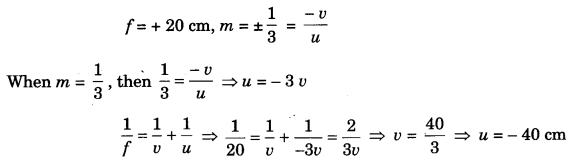Therefore the mirror is convex and the image is virtual, erect, and diminished.Therefore, the mirror is concave and the image is real, inverted and diminished.

Question 4.
A student focussed the image of a candle flame on a white screen using a convex lens. He noted down the position of the candle screen and the lens as under  (NCERT Exemplar)
Position of candle = 12.0 cm
Position of convex lens = 50.0 cm
Position of the screen = 88.0 cm
(i) What is the focal length of the convex lens?
(ii) Where will the image be formed if he shifts the candle towards the lens at a position of 31.0 cm?
(iii) What will be the nature of the image formed if he further shifts the candle towards the lens?
(iv) Draw a ray diagram to show the formation of the image in case (iii) as said above.
(i) Here, u = – 38 cm, v = 38 cm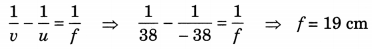(ii) u = – 19 cm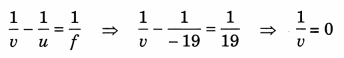V = ∞

(iii) The object (candle) will be between the principal focus and optical centre. Hence image formed will be enlarged, virtual and erect.

(iv)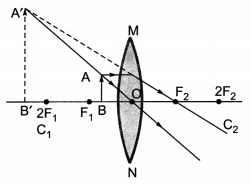Question 5.
Identify the device used as a spherical mirror or lens in the following cases, when the image formed is virtual and erect in each case.
(a) Object is placed between device and its focus, image formed is enlarged and behind it.
(b) Object is placed between the focus and device, image formed is enlarged and on the same side as that of the object.
(c) Object is placed between infinity and device, image formed is diminished and between focus and optical centre on the same side as that of the object.
(d) Object is placed between infinity and device, image formed is diminished and between pole and focus, behind it.
(a) Concave mirror
(b) Convex lens
(c) Concave lens
(d) Convex mirror

Question 6.
Sudha finds out that the sharp image of the window pane of her science laboratory is formed at a distance of 15 cm from the lens. She now tries to focus the building visible to her outside the window instead of the window pane without disturbing the lens. In which direction will she move the screen to obtain a sharp image of the building? What is the approximate focal length of this lens? (NCERT Exemplar)Sudha should move the screen towards the lens so as to obtain a clear image of the building. Here the object is at infinity and hence a sharp image of the object is formed at the principal focus (F1) of the lens. Hence, the approximate focal length of this lens will be 15 cm.

### Extra Questions for Class 10 Science Chapter 10 Value Based Questions

Question 1.
Amit and Sumeet visited National Bal Bhawan on Environmental day. There they saw a concentrator type of solar cooker installed for Basic heating usage. Give the answers of the following questions:
(i) Type of mirror used in concentrating type solar cooker
(ii) Values shown by the organisation